Publicité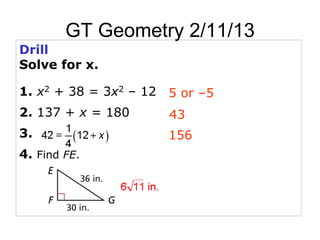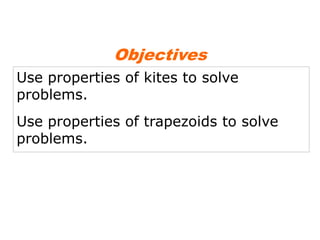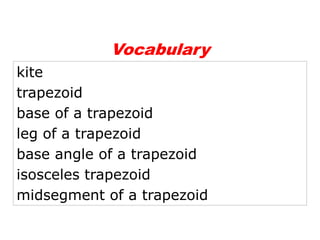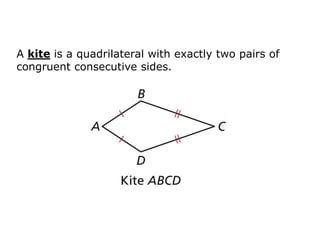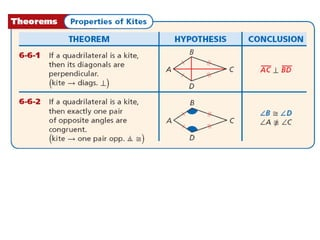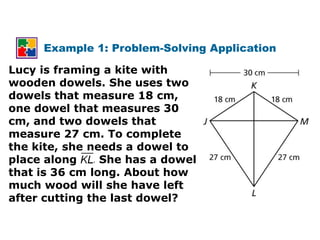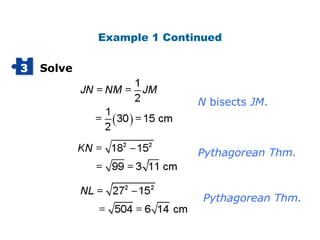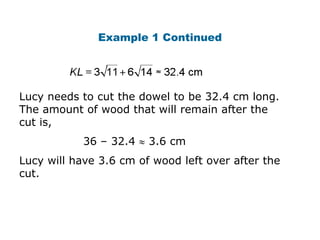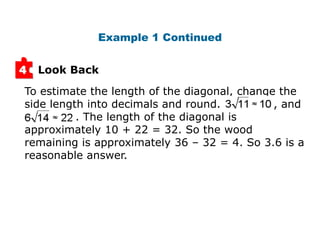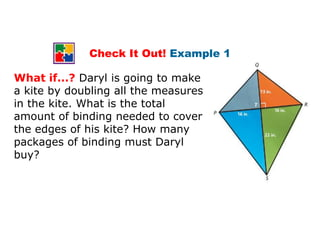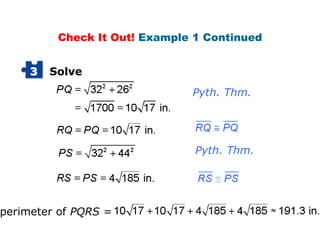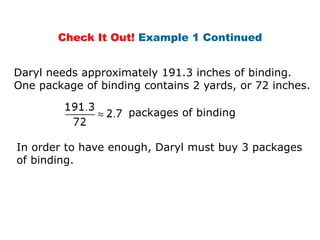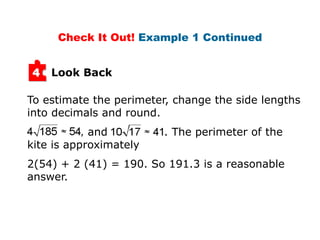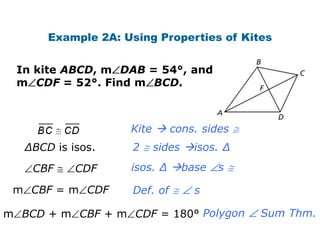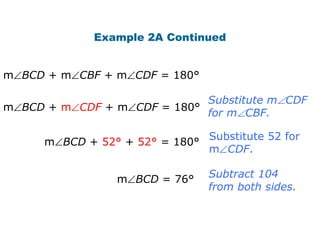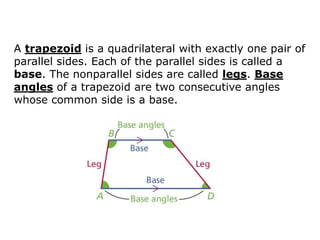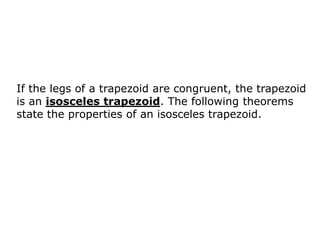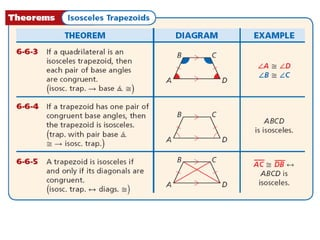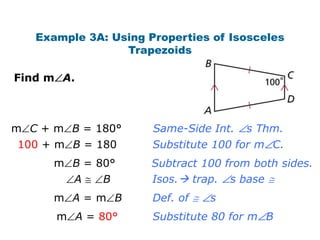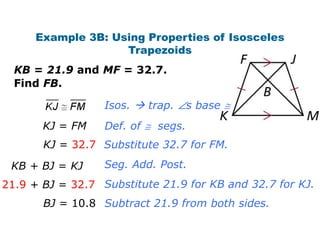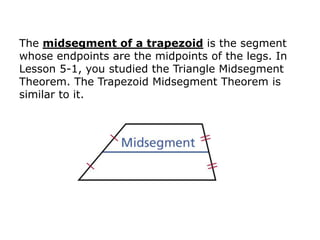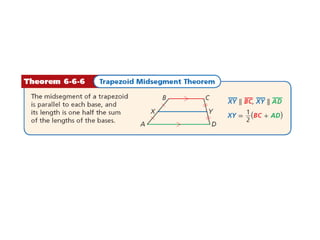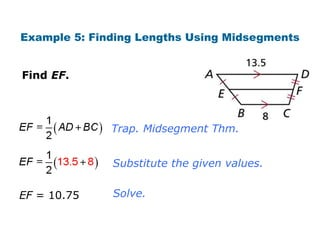1 sur 23
Publicité

### Traps and kites updated2013

1. GT Geometry 2/11/13 Drill Solve for x. 1. x2 + 38 = 3x2 – 12 5 or –5 2. 137 + x = 180 43 3. 156 4. Find FE.
2. Objectives Use properties of kites to solve problems. Use properties of trapezoids to solve problems.
3. Vocabulary kite trapezoid base of a trapezoid leg of a trapezoid base angle of a trapezoid isosceles trapezoid midsegment of a trapezoid
4. A kite is a quadrilateral with exactly two pairs of congruent consecutive sides.
5. Example 1: Problem-Solving Application Lucy is framing a kite with wooden dowels. She uses two dowels that measure 18 cm, one dowel that measures 30 cm, and two dowels that measure 27 cm. To complete the kite, she needs a dowel to place along . She has a dowel that is 36 cm long. About how much wood will she have left after cutting the last dowel?
6. Example 1 Continued 3 Solve N bisects JM. Pythagorean Thm. Pythagorean Thm.
7. Example 1 Continued Lucy needs to cut the dowel to be 32.4 cm long. The amount of wood that will remain after the cut is, 36 – 32.4 3.6 cm Lucy will have 3.6 cm of wood left over after the cut.
8. Example 1 Continued 4 Look Back To estimate the length of the diagonal, change the side length into decimals and round. , and . The length of the diagonal is approximately 10 + 22 = 32. So the wood remaining is approximately 36 – 32 = 4. So 3.6 is a reasonable answer.
9. Check It Out! Example 1 What if...? Daryl is going to make a kite by doubling all the measures in the kite. What is the total amount of binding needed to cover the edges of his kite? How many packages of binding must Daryl buy?
10. Check It Out! Example 1 Continued 3 Solve Pyth. Thm. Pyth. Thm. perimeter of PQRS =
11. Check It Out! Example 1 Continued Daryl needs approximately 191.3 inches of binding. One package of binding contains 2 yards, or 72 inches. packages of binding In order to have enough, Daryl must buy 3 packages of binding.
12. Check It Out! Example 1 Continued 4 Look Back To estimate the perimeter, change the side lengths into decimals and round. , and . The perimeter of the kite is approximately 2(54) + 2 (41) = 190. So 191.3 is a reasonable answer.
13. Example 2A: Using Properties of Kites In kite ABCD, m DAB = 54°, and m CDF = 52°. Find m BCD. Kite  cons. sides ∆BCD is isos. 2 sides isos. ∆ CBF CDF isos. ∆ base s m CBF = m CDF Def. of s m BCD + m CBF + m CDF = 180° Polygon Sum Thm.
14. Example 2A Continued m BCD + m CBF + m CDF = 180° Substitute m CDF m BCD + m CDF + m CDF = 180° for m CBF. Substitute 52 for m BCD + 52° + 52° = 180° m CDF. m BCD = 76° Subtract 104 from both sides.
15. A trapezoid is a quadrilateral with exactly one pair of parallel sides. Each of the parallel sides is called a base. The nonparallel sides are called legs. Base angles of a trapezoid are two consecutive angles whose common side is a base.
16. If the legs of a trapezoid are congruent, the trapezoid is an isosceles trapezoid. The following theorems state the properties of an isosceles trapezoid.
17. Example 3A: Using Properties of Isosceles Trapezoids Find m A. m C + m B = 180° Same-Side Int. s Thm. 100 + m B = 180 Substitute 100 for m C. m B = 80° Subtract 100 from both sides. A B Isos. trap. s base m A=m B Def. of s m A = 80° Substitute 80 for m B
18. Example 3B: Using Properties of Isosceles Trapezoids KB = 21.9 and MF = 32.7. Find FB. Isos.  trap. s base KJ = FM Def. of segs. KJ = 32.7 Substitute 32.7 for FM. KB + BJ = KJ Seg. Add. Post. 21.9 + BJ = 32.7 Substitute 21.9 for KB and 32.7 for KJ. BJ = 10.8 Subtract 21.9 from both sides.
19. The midsegment of a trapezoid is the segment whose endpoints are the midpoints of the legs. In Lesson 5-1, you studied the Triangle Midsegment Theorem. The Trapezoid Midsegment Theorem is similar to it.
20. Example 5: Finding Lengths Using Midsegments Find EF. Trap. Midsegment Thm. Substitute the given values. EF = 10.75 Solve.
Publicité